This video was brought to you by GeneralPAC.com, making power systems Intuitive, Open and Free for Everyone, Everywhere. Consider subscribing and supporting through patreon.com/GeneralPAC. This is a mechanism for you to support us financially so we can continue making high quality power system video tutorials. Our corporate sponsor for this topic is AllumiaX.com from Seattle, Washington. Contact them for industrial and commercial power system studies.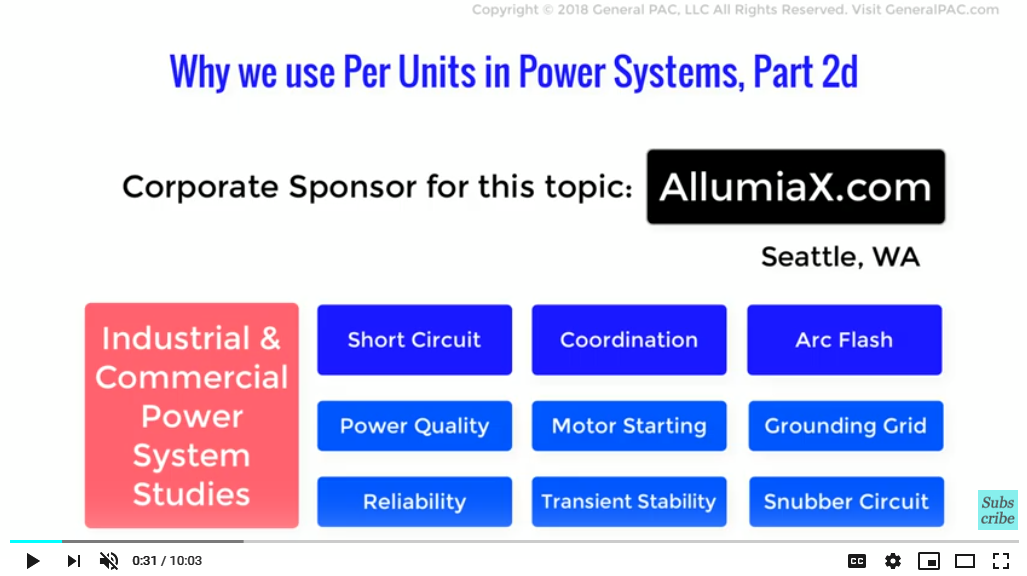Why we use Per-Units in Power Systems, Part 2d.

In this video, we will demonstrate another advantage of using Per-units in Power System calculations. This aspect is an extension of the previous video.

In a power system, multiple transformers result in multiple voltage levels at different points in the system. Per-unit calculations ease the analysis of such systems by eliminating these multiple voltage levels and bringing the impedances on both sides of the transformer on a single scale. We demonstrated this aspect in detail in Part 2c, with a single transformer. Here in Part 2d we will consider a power system with more than one transformer, thus tackling multiple voltage levels.

And for this, we will take an example from the book Power System Analysis by John J. Grainger (International Edition 1994).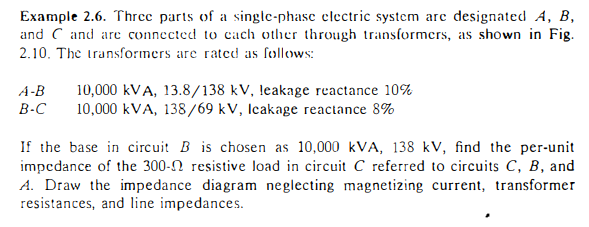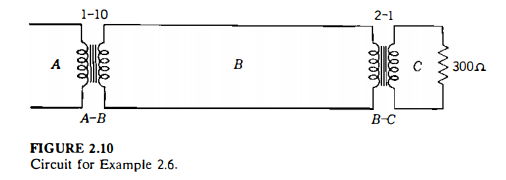Example 2.6, pg. 57, Power System Analysis by John J. Grainger (International Edition 1994)

So, let’s solve this question. Keep in mind this is a single phase system so the base impedance calculations are different as compared to a three phase system.

Since we are given the resistance in circuit C, we’ll solve for per unit value of that resistance at the base voltage of 69 kV. Circuit C also has a base value of power of 10,000 kVA so the base value of impedance becomes as follows.

Z c,base =
Vc,base 2 / Sc,base
=
(69 KV)2 / 10,000 KVA
= 476 Ω
Z c,p.u =
Zc,actual / Zc,base
=
300Ω / 476Ω
= 0.63 p.u

Now, based on part 2c of this series, we can stop here as we already know the per unit impedance for both transformers and the load. The load pu impedance was calculated as 0.63pu and since the transformer leakage reactance was given as a percentage in the problem statement, we know they corresponds to per unit values. So the equivalent circuit of this problem looks like this: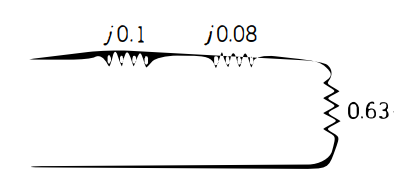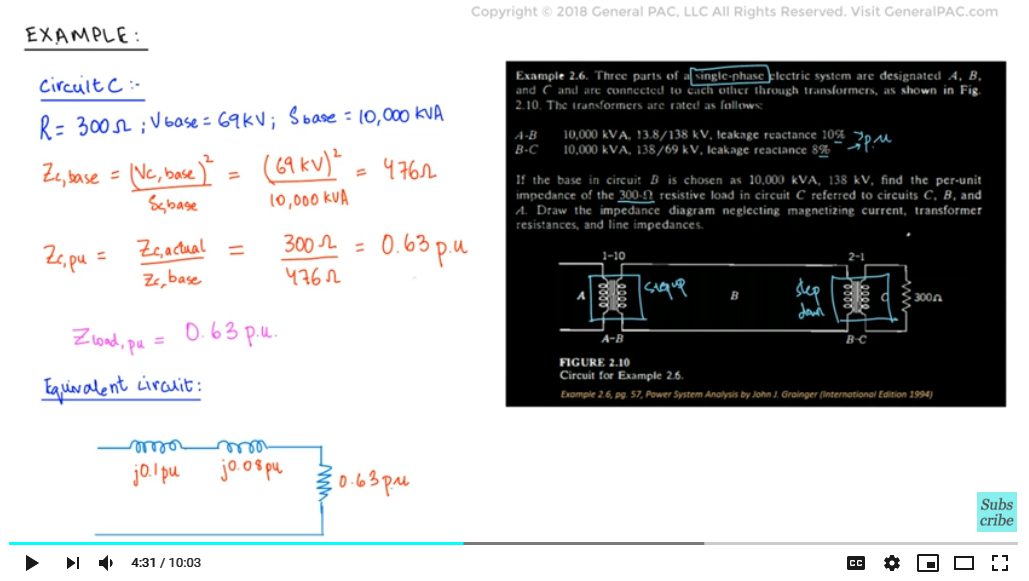Despite solving this answer, we will go through and show the advantage of using per units.

Because the selection of base in various regions of the system is determined by the turns ratio of the transformers, and because the base KVA is the same in all regions of the system, the per unit impedance of the load referred to any regions of the system will be the same. This will be illustrated in the remaining part of this tutorial.

We have calculated the per unit impedance of the circuit referred to Circuit B, and we also know that the per unit impedance in Circuit B would be equal to the per unit impedance in Circuit C i.e. 0.63 p.u. as described in Part 2c.

We first find out the base value of impedance in Part B of the circuit by taking 138 kV as the base value of voltage and the base value of power remains the same at 10,000 kVA

Z B,base =
VB,base 2 / SB,base
=
(138 KV)2 / 10,000 KVA
= 1904.4 Ω

Now if we were to calculate the actual value of impedance in circuit B we would multiply it with the square of turns ratio of 2:1 and the actual value is 1200 ohms.

Z B,actual = 300Ω ×
(2/1)2
=1200 Ω

Lastly when we convert the actual value to P.Us we find that it comes out to be essentially the same. And here is how:

Z B,p.u =
ZB,actual / ZB,base
=
1200 / 1904.4
= 0.63 p.u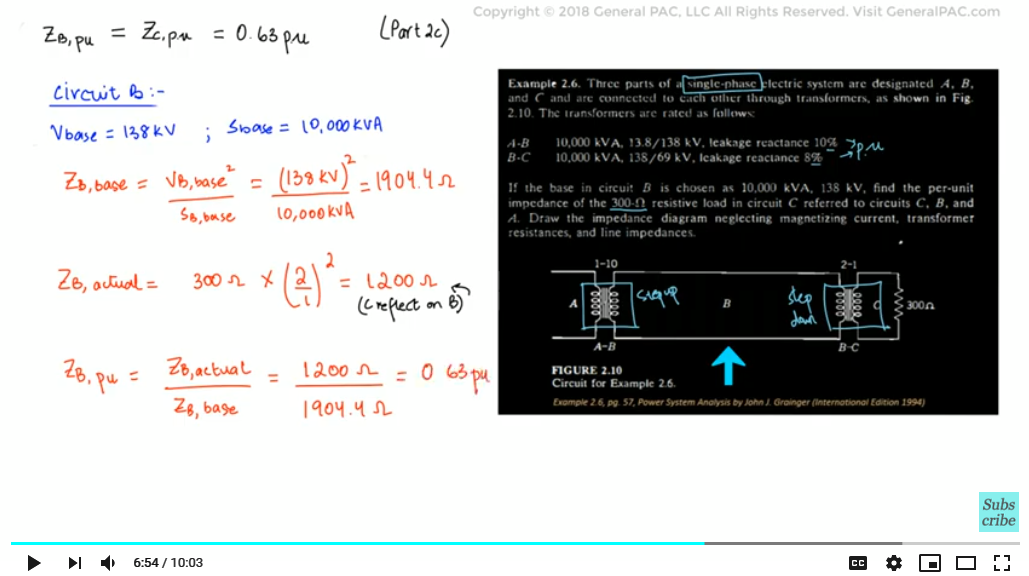So, here we have proved what we learnt in Part 2c… which is that per unit impedance on HV side and LV side of the transformer will be exactly the same just like how Z_(C,p.u ) and Z_(B,p.u )are the same.

Now if we notice, we can see that Circuits A and B are the primary and secondary sides of the first transformer, respectively. Logic says that the per unit impedance of Circuit A will be equal to that of Circuit B. Let’s find out if that statement is correct.

Z A,p.u =
VA,base2 / SA,base
=
(13.8kV)2 / 10,000kVA
= 19 Ω
Z A,actual =
1200Ω × / (1/10)2
=12 Ω
Z A,p.u =
ZA,actual / ZA,base
=
12Ω / 19Ω
= 0.63 p.u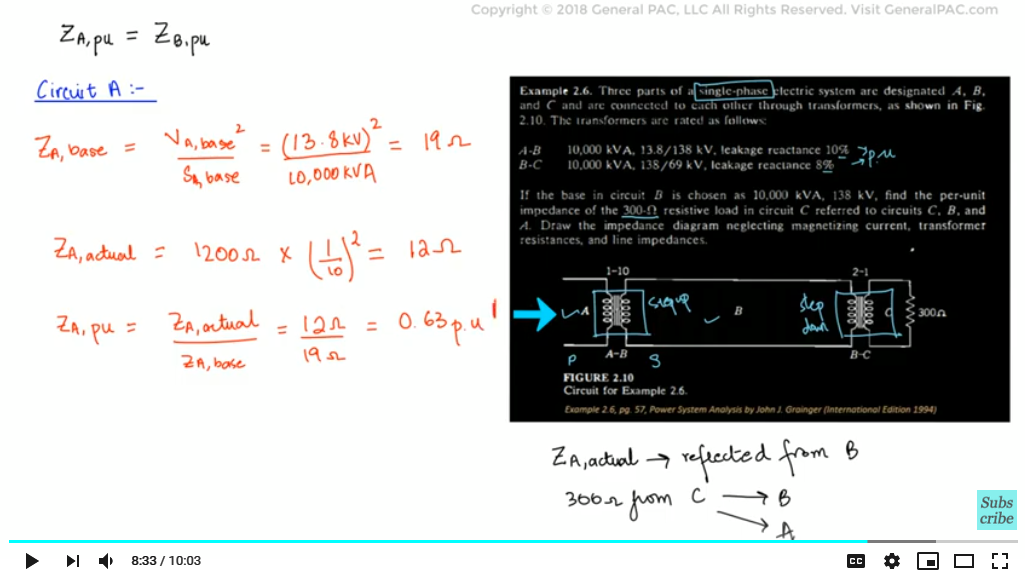Finally, we have the per unit impedances in all parts of the circuit in the power system of this example. It equals to 0.63 p.u in all parts of the circuit which reflects that when a power system consists of more than one transformer, the per unit impedance for every side of every transformer remains the same. We can draw the equivalent circuit like this:Per-unit equivalent circuit for the above example

Notice that the multiple voltage levels are eliminated by making use of Per-units and the circuit consists of just impedances that have been reduced to a single voltage level that is common to the entire system.

We can also conclude that no matter how many transformers and voltage levels are added in a system, we can find the impedance by simply doing calculation for only one side of one transformer. See! How per unit calculations can drastically reduce the mathematics involved.

In the next video, we will be dealing with an example for per unit systems in three-phase systems calculations.

We hope, you have a continued interest in this topic and series as a student or professional. We also hope you find this content useful and enlightening. Please consider subscribing to GeneralPac.com or becoming a patron on patreon.com.

GeneralPAC.com. Making Power Systems Intuitive and free to everyone, anywhere, everywhere.

Thankyou.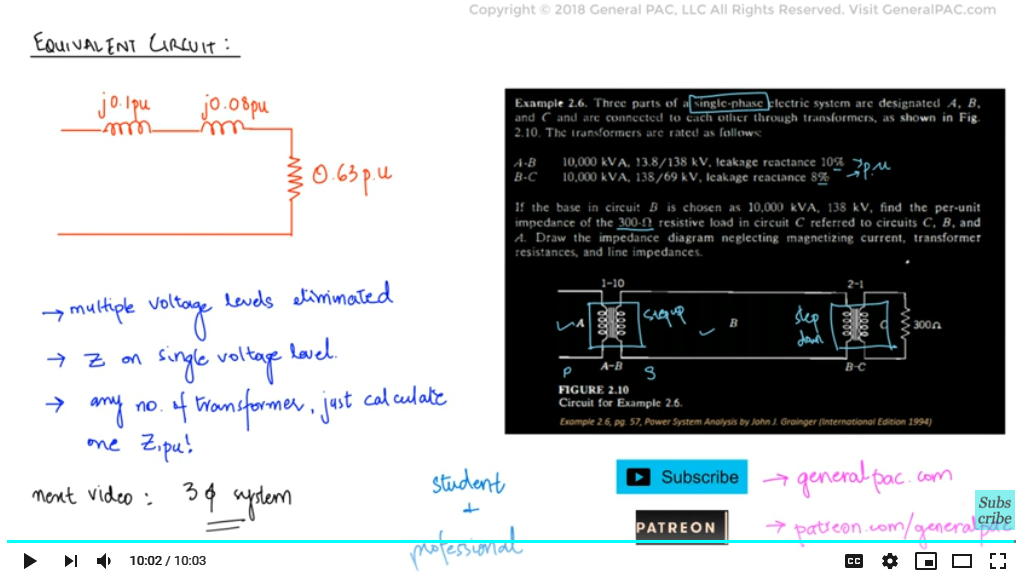#### Greetings from the GeneralPAC Team!

We make high-quality Power Systems Video Tutorials on complex topics that are free and open to everyone!  Thank you so much for supporting us through Patreon so we can continue doing good and valuable work.

What is Patreon and why do we use it?

Patreon is a fantastic portal that allows our fans and community to make monthly contribution (like Netflix subscription) so we can continue creating high-quality power systems video tutorials. In return, you get access to incredible perks like voting on future topics, getting your questions answered, access to VIP Q/A webinars with the creators of GeneralPAC, and much more! We THANK YOU for supporting us

Why do we need your support?

An incredible amount of time and effort is needed to develop high-quality video tutorials. Each video (Part 1 for example) takes approximately 10 hours to complete which includes learning the concept ourselves, brainstorming creative ways to teach and explain the concepts, writing the script, audio recording, video recording, and editing. It's no wonder why Hundreds-of-Thousands of people have watched, liked, subscribed, and left positive comments on Youtube channel. Your support truly makes all the difference.